# Numerical Simulation of Phase Transformation and Reorientation in Single Crystalline Shape Memory Alloys

JJ Zhu1*, NG Liang2, M Cai3, KM Liew4 and WM Huang5

1School of Civil Engineering and Architecture, Wuyi University, China

2LNM Institute of Mechanics, Chinese Academy of Sciences, Beijing, China

3Department of Engineering Technology, University of Houston, USA

4Department of Architecture and Civil Engineering, City University of Hong Kong, Kowloon, Hong Kong

5School of Mechanical and Aerospace Engineering, Nanyang Technological University, Singapore

Received Date: September 08, 2018;  Published Date: September 24, 2018

#### Abstract

In our previous paper , a constitutive model was developed for the stress-induced martensitic transformation and reorientation in single crystalline shape memory alloys. The critical condition and evolution equation for the phase transformation and reorientation were proposed. In this paper, the model proposed in our previous paper  is applied to simulate the behavior of stress-induced phase transformation in a CuZnAl single crystal and reorientation between CuAlNi martensite lattice correspondence variants. It is found out that the prediction results are consistent with the experimental data reported in the literature.

Keywords: Phase transformation; Thermomechanical processes; Microstructure; Constitutive behavior; Shape memory alloy

#### Introduction

Shape memory alloys have been widely used in mechanical, automotive, aerospace, nuclear, dental, medical and domestic appliance industries [2-5]. In order to provide a robust computation tool for engineers, much attention has been devoted to model the mechanical behavior of shape memory alloys [6-41]. However, the sophisticated thermo-mechanical behaviors under various loading conditions make the modeling a complex task. Under external mechanical/thermal loading, shape memory alloys exhibit some unique phenomena of practical interest, such as, superelastic behavior and shape memory effect. Those phenomena are due to the phase transformation and reorientation at microscopic level. Basic transition process comprises of forward transformation from austenite to martensite habit plane variant, reverse transformation from martensite habit plane variant to austenite, and reorientation among martensite lattice correspondence variants. Experimental studies on phase transformation, e.g. [42,43] and reorientation, e.g.  of single crystalline shape memory alloys were reported.

In our previous paper , a 3-D thermodynamic model for constitutive behavior of single crystalline shape memory alloys was developed. The model is based on the fact that martensite lattice correspondence variants are energy potential wells. Internal interaction energy among austenite and martensite variants is estimated by generalized Mori-Tanaka theory. We also derived the thermodynamic driving forces corresponding to the forward/ reverse phase transformation and reorientation, and the critical condition and the evolution equation for phase transition and reorientation. Moreover, the complex interior hysteresis loop is presented by the jump of critical thermodynamic driving force. In this paper, the model proposed in our previous paper  is used to investigate the behaviors of both stress-induced and thermally-induced phase transformation in a CuZnAl single crystal and martensite reorientation between CuAlNi martensite lattice correspondence variants.

#### Phase Transformation

Experimental data of forward and reverse martensitic transformations of some single crystalline shape memory alloys are available in the literature. For example, the uniaxial tensile tests of CuZnAl single crystals (transformation from DO3 austenite to 18R martensite upon straining) were reported [42,43]. It was assumed that only one martensite habit plane variant was induced in this process. Note that the induced martensite habit plane variant is the one in favor by the applied actual stress state. In this material, the number of habit plane variant, H=24. Each habit plane variant is a lattice correspondence variant. Thus, the transformation eigenstrain of the habit plane variant is the same as the eigenstrain of lattice correspondence variant and Eq. (57) in our previous paper  is valid. Eqs. (38) & (57) in our previous paper  yieldwhere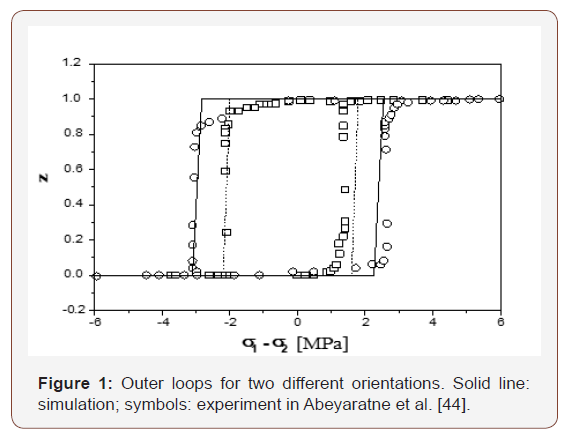The first term of Eq. (2) stands for the interaction energy between martensite and austenite, while the second term for the interaction energy stored in the interface among martensite variants. Muller and Huo [43,45] have demonstrated that if two phases are mixed randomly, the number of interfaces per unit volume is proportional to z(1− z) , where z is the volume fraction of one phase. Figure 1 in Huo and Muller  shows that the relationship between the number of inter-phase and martensite volume fraction z is parabolic. The peak is located at z = 1/ 2 . If the elastic energy stored in per unit area of inter-phase is assumed to be a constant, the interaction energy can be expressed as , where A is a material constant. In trilinear model, for instance, [43,46], constant A is defined to be proportional to the slope of the second line, which corresponds to the phase transformation range (refer to Figure 2 in , Figures 3 & 4 in ). Thus, the mean elastic energy stored in the inter-phase at micro level is linked to the macro behavior by A . As pointed out in Seelecke , elastic distortion energy stored in the twinned plane is much less than the inter-phase (between austenite and martensite) energy stored in habit plane. If only the first term of the right side of Eq. (2) is reserved, one has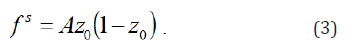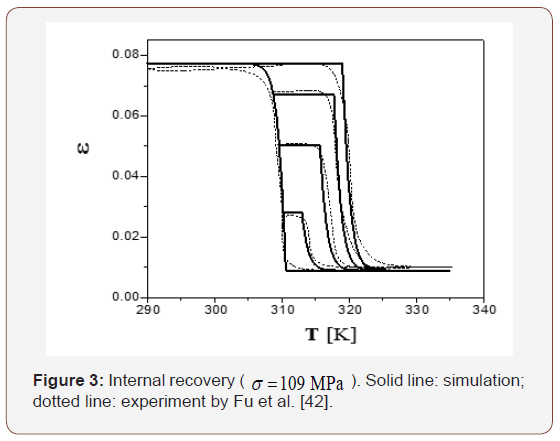Substitution of Eq. (3) into Eq. (1) yields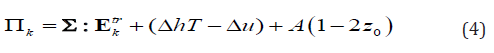Supposing that the tensile axis is along direction S, the applied stress may be expressed asHere, we consider a particular case, assuming that the external load (mechanical and/or thermal load) has no apparent change during the phase transition, so that only one martensite habit plane variant (in the favorable orientation of the applied stress) is produced. From Eq. (4), the thermodynamic driving force corresponding to the active habit plane variant, which is in favor by the applied stress is reduced to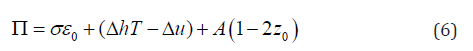and the recoverable strain along the tensile axis is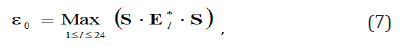According to our previous paper , the critical condition for the start of the phase transformation is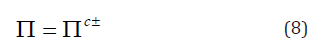and the phase transformation evolution equation can be expressed asOnce the nucleation starts from the forward transformation, Πc− moves back to its maximum value (Π0 ) instantly. While when the reverse transformation starts, Πc+ returns to its minimum value ( Π0+). It is also reasonable to assume that Π0+ = Π0- = Π0.

In the tensile experiment on CuZnAl single crystals by Fu et al. , the intersection gauge area is 4.5×10−6m2 , the gauge length is 3.4×10−2m, the mass is 1.185×10−3 kg. If the International System of Units (SI) is adopted, vertical scale in figures of Fu et al. 42 should be divided by 4.5×10−6m2 and the horizontal scale should be divided by 28.7 m/kg. Based on the data in Fu et al. , we take MPa Π0= 0 for this particular sample. From Figure 5 of Fu et al. , one can obtain [Refer to page19 of  for details],As shown in Eq. (4.3) of Fu et al. , the inside area of a full hysteresis loop is 2A. Assume that the stress at points B and E are σB and σE , respectively, in Figure 5 (so as in Figure 2 & 6), then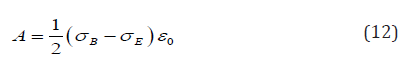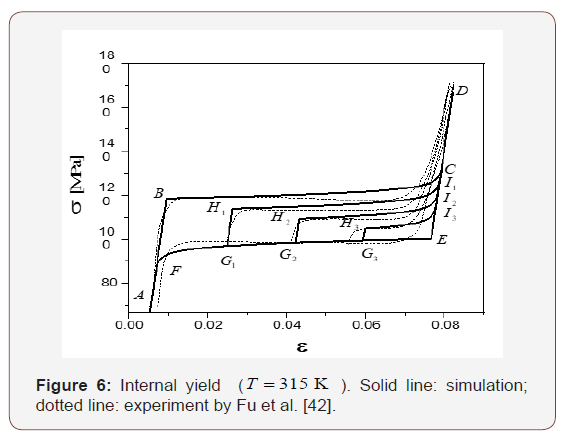For simplicity, A can be directly obtained from Fu et al.  as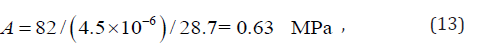Δu and Δh may be determined by the hysteresis loops at different temperatures. For example, B in Figure 5 is the starting point of the forward transformation. Thus, we have Π = 0 and z0 =1 at point B . Substituting them into Eq. (6) yields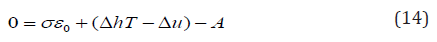Two hysteresis loops at two different temperatures, T1 and T2 , can be obtained experimentally (as shown in Figure 2 of ). Thus,where σ1 and σ2 are stresses for point B at temperature T1 and T2 . From Eqs. (15) and (16)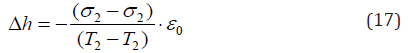and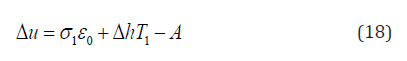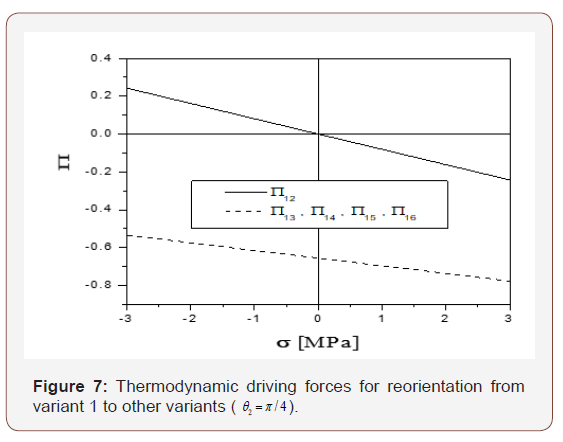Using the data in Fu et al.  (refer to Figure 7 and Eq. (3.31) in  for details), we have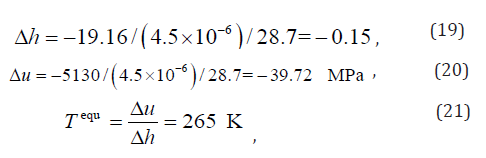Note that (λ − A) is the parameter for hardening; in which λ > A stands for hardening, λ < A stands for softening and λ = A corresponding to a horizontal plateaus. All three cases are possible depending on the exact material and the loading/unloading conditions. We show horizontal plateaus in Figures 2,5 & 6 and small hardening in Figures 3,8 & 9 (also referring to page 5 of  for details]. We assume a low level of hardening (1% ) in our simulation for simplicity, i.e. (λ − A) = A×1%. Thus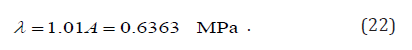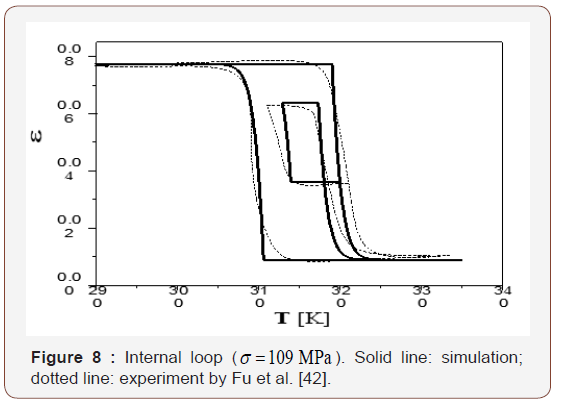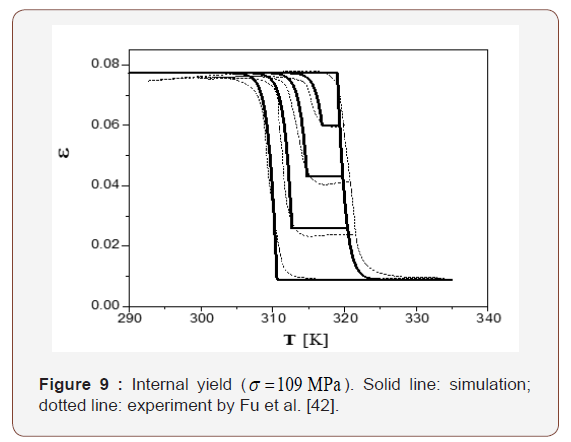We also introduce parameter μ to describe the “turn-up tail” behavior when the transformation nearly finishes, and make an adequate assumption μ = A×5% i.e.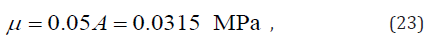The CuZnAl single crystal exhibits superelastic behavior in the isothermal process at T = 315 K in . Three different types of cyclic stress-strain curves were reported. The simulation of present model against that experiment are plotted in Figures 2,5&6. As shown in Figure 5, the whole material is austenite at point A . When loaded to point B , the thermodynamic driving force Π reaches the critical value Π0+ (= 0). Consequently, the forward transformation starts. Loading from B to C , both Π and Πc+ increase and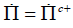. The evolution of the forward phase transformation can be determined by integrating Eq. (9). When loaded to point C , the whole material transforms into 100%martensite. The material deforms elastically from C to Dduring loading and from Dto E upon unloading. When unloaded to point E , the thermodynamic driving force Π decreases to the critical value, Π0- (= 0), and the reverse transformation starts. Eq. (9) is also employed for modeling the evolution of the reverse phase transformation. Upon unloaded to point F , the whole material transforms back to austenite. A-BC- D-E-F-A is a complete forward-reverse transformation loop. In another loading path A-B-G1-H1-I1-A, the forward transformation starts upon loaded to B . Upon loading to point G1 only a part of the material transforms into martensite. Unloading from G1 to H1 , because Πc−< Π < Πc+, the material deforms elastically (note that during this process, Πc+ maintains its value as at point G1). When unloaded to point H1 the thermodynamic driving force Π reaches the critical value, Π0- (= 0). Then the reverse transformation starts. When unloaded to point I1, the whole material transforms back to austenite. Thus, A-B-G1-H1-I1-A is an incomplete forwardreverse transformation path. Similarly, loops of A-B-G2-H2-I2-A and A-B-G3-H3-I3-A illustrate two incomplete forward-reverse transformation paths. Points are practically lie on a diagonal line, which corresponds to Π = 0 .

In Figure 2, A-B-C-D-E-F-A is a complete forward-reverse transformation. In the loop of A-G-H-I-J-K-D-E-F-A, Π increases to Πc+ = Π0+(=0) upon loading to B . Thus, the forward transformation starts from point B . During loading from B to G , Πc+ increases at the same rate as that of Π in the evolution of forward transformation. The deformation is elastic during unloading from G to H and Πc+ remains the value as at point G . At point H , Π is reduced to Πc- = Π0-(=0). Thus, the reverse transformation starts and Πc+ reverses back to the minimum Πc- = Π0-(=0). Similar to the forward transformation, Πc− decreases from H to I at the same rate of Π in the evolution of the reverse transformation. Reloading from I to J , the material deforms elastically and Πc− maintains the value as at point I . At point J , Π increases to Πc+ = Π0+(=0). The forward transformation restarts and Πc− returns to the maximum Πc- = Π0-(=0). Upon loading to K , the whole material transforms into martensite again. The points B, J ,H, E lie on a diagonal line, which corresponds to Π = 0.

Temperature plays a critical role in mechanical response of shape memory alloys. Three different types of cyclic temperature vs. strain curves of a CuZnAl single crystal under a constant stress of σ = 109 MPa were reported in . It was found that the material exhibits superelastic behavior under those loading conditions, and the effect of change in temperature on the transformation is equivalent to the change of stress. Equilibrium of σ and T is associated with a Clapeyron-like equation, which is analogous to the Clausius-Clapeyron equation. Differentiating Eq. (6) and keeping Π as a constant, one hasBoth analytical result and experiment data of this case are shown in Figures 3,8 & 9. Comparing Figures 2,5&6 with Figures 3,8 & 9, we notice that the constant-stress process is similar to the constant-temperature process. However, in the constant-stress process, the prescribed stress must be large enough to ensure only one martensite variant is produced. Therefore, the associated deformation is always super elasticity.

According to Eq. (6), there are three parts included in the thermodynamic driving force Π for the forward (or reverse) transformation. The first is mechanical load device, the second is temperature load device, and the third is the stored energy. The sum of the first two is also called load device energy. It can be seen from Figures 2,3,5,6,8&9 that the variation of load device energy between the forward and the reverse transformation is about 1.5 MPa . The variation of stored energy is about 1.26 MPa . Both are of the same order of magnitude. Thus, the stored energy between austenite and martensite phase-interface cannot be ignored.

It appears that our simulation is very close to the measured behavior.

#### Reorientation

We now consider the reorientation between 2H martensite variants in a Cu-14.0wt%Al-3.9wt%Ni shape memory single crystal. Experimental data are taken from Abeyaratne et al. . 2H martensite of CuAlNi has 6 corresponding variants and 24 habit plane variants [48-50]. Each habit plane variant consists of two twin-related lattice correspondence variants.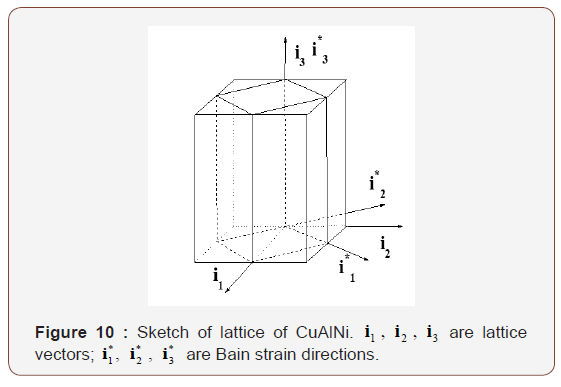Suppose that the lattice coordinate system of 3 DO austenite is o − i1 i2 i3 and the principle directions of Bain strain are along the axes of coordinate system o − i1* i2* i3* (Figure 10). In the coordinate system o − i1 i2 i3 , six Bain strains can be expressed by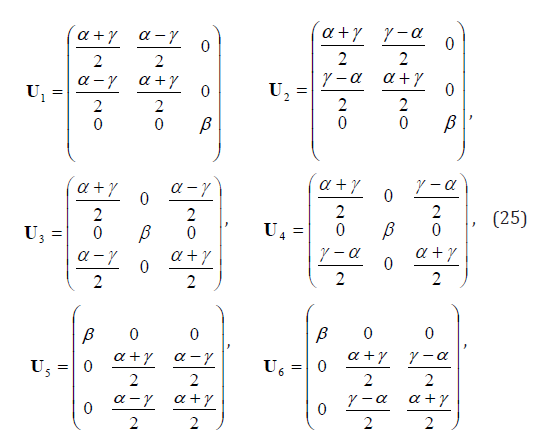where α , β , γ have been measured by Otsuka and Shimizu  asThe corresponding Green strain is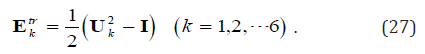According to Abeyaratne et al. , initially the single crystal is martensite variant 2. Stresses were applied along two orthogonal directions 1 S and 2 S , i.e.,where θ is the angle between S1 and i1 . In the experiment, tw θ were chosen, namely, π/8(denoted by θ1) and π/4 (denoted by θ1 ). Thus, the applied stress may be expressed as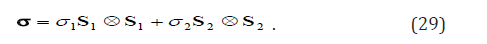Variant 2 may transform into variants 1,3,4,5 or 6 upon stressing. The corresponding process variables are ζ21, ζ 23, ζ24 , ζ25, and ζ24, and their stoichiometric coefficients are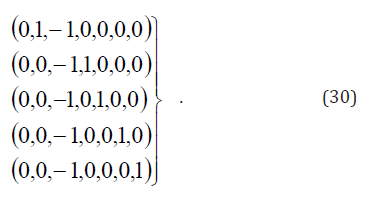Only two martensite lattice correspondence variants were presented in . The volume fraction of austenite, z,0 = 0 . In this case, Eq. (2) is reduced to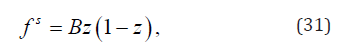where is the volume fraction of one variant, while (1− z) is another.

Elastic energy stored in the twin-interface between the martensite lattice correspondence variants can be evaluated by the number of needle tips . It was found that the density of needle tips terminated in transition layer is mainly dependent on the volume fraction of martensite lattice correspondence variant. Total transition layer energy can be written as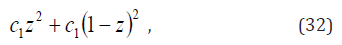where c1 and c2 are constants standing for two types of transition layers observed. Since there is no other difference between these two martensite lattice correspondence variants except for the orientation, c1 and c2 should be the same. If c1 = c2 , with the addition of a constant energy term, Eq. (32) may be rewritten as Bz(1− z) . Based on the non-local interaction theory, Rogers also concluded that the interaction energy between two martensite variants may be expressed by Bz(1− z) . According to Abeyaratne et al.  onstant B is about 0.017MPa . From Figure 9 of , the variation of load device energy between reorientation and its reverse process is about 0.15MPa . Thus, transition layer energy is ignorable as compared with load device energy, i.e., Eq. (3) is an acceptable approximation for martensite variant reorientation.

Using Eq. (65) in our previous paper1 (or substituting Eq. (30) into Eq. (58) in our previous paper ) and ignoring interaction energy, the corresponding thermodynamic driving forces can be written as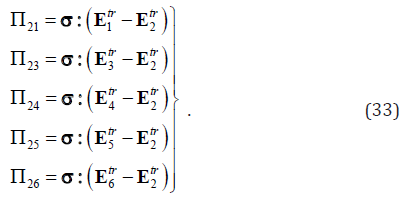From Eqs. (25)-(29), we have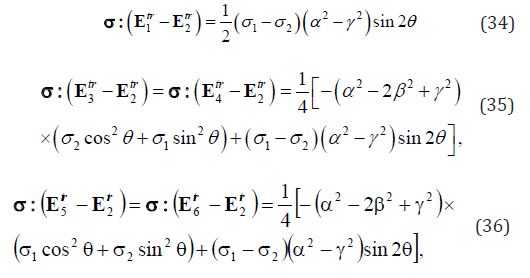Thus,Let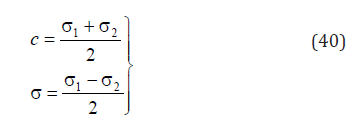Hence,According to Abeyaratne et al. , σ1 +σ =10.7MPa , and −1.5 MPa ≤σ ≤1.5MPa . Substituting Eq. (26) into Eqs. (37)- (39) and assuming θ = Π/8 and , c = 5.35MPa the variation of the thermodynamic driving force against increases from -1.5 MPa to 1.5 MPa. As shown in Figure 11, Π21 is much larger than Π23 and Π24 . Hence, under the given loading condition described in , variant 2 has no other choice but transforms into variant 1.Upon further loading, variant 1 may transform to variants 2, 3, 4, 5 or 6. Introducing process variables ζ12 , ζ1321 , and ζ15 , their corresponding stoichiometric coefficients (ν0l1l2l …ν6l) ( l = 2, 3, 4, 5, 6) are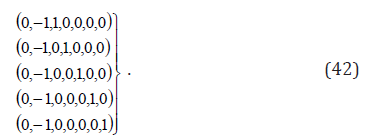The corresponding thermodynamic driving forces can be written asEqs. (25)-(29) yieldThusSubstituting Eq. (26) into Eqs. (47)-(49) and assume θ1 = π/8 and c = 5.35 MPa , the relation between the thermodynamic driving force vs. σ (which decreases from 1.5 MPa to -1.5 MPa) can therefore be obtained. As shown in Figure 12, 12 Π is much larger than Π13 and Π15 . Thus, variant 1 is only able to transform into variant 2 first under this loading condition, just like that described in .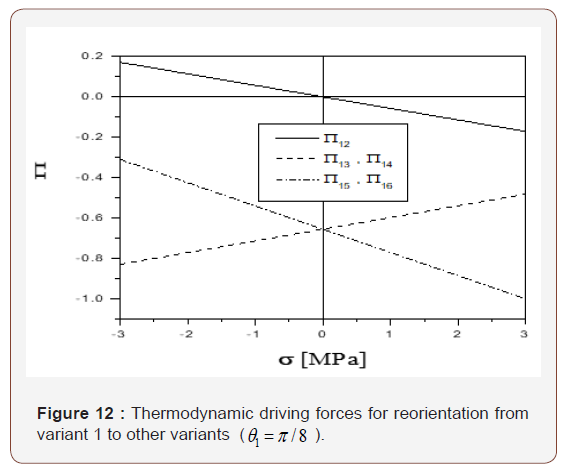Similar results can be obtained for the case of θ2 = π/4, as showed in Figures 4 & 7.

The critical condition for reorientation from variant 2 to variant 1 is given by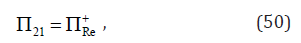while the critical condition of reorientation from variant 1 to variant 2 is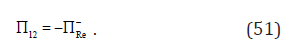Because they are complimentary forward and reverse processes, Eqs. (50) & (51) may be written together as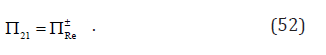Substitution of Eqs. (33) & (37) into Eq. (52) yields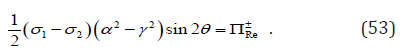When θ = π/4, according to Figure 2(b) of Abeyaratne et al. , we haveTheoretically, orientation is the only difference between variant 1 and variant 2. Thus, ΠRe+ = −ΠRe = ΠRe0. The observed non-symmetry in the forward and reverse reorientation between variant 2 and variant 1 might be due to the instrumental errors in testing or specimen preparation.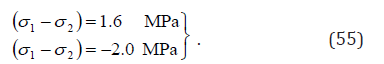The result of Eq. (55) is plotted in Figure 13 against the experimental data (i.e., Figure 3 in Abeyaratne et al., 1996).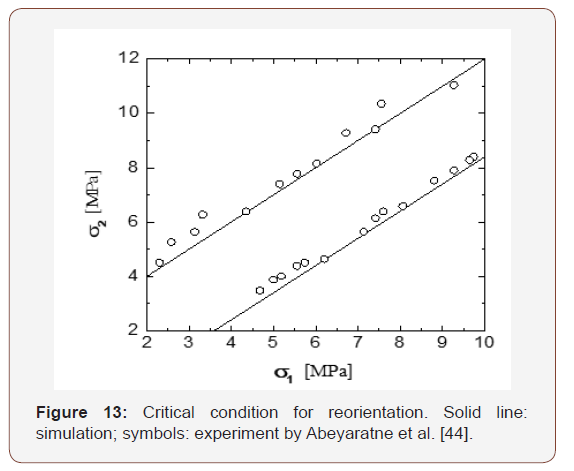Suppose that the volume fraction of variant 1 is z , according to Eq. (77) in our previous paper , the evolution equation for reorientation can be written aswhere λ1 can be determined from Figure 9 of Abeyaratne et al. :In the case of θ2 = π/8, similar result can be derived. Let 1 2 σ − σ vary between − 6.0 MPa and 6.0 MPa , the change of volume fraction of variant 1 vs. σ1 σ2 is shown in Figure 14. This is consistent with the experimental result in .

ΠRe+, ΠRe and λ1 in Eqs. (54) & (57) can be determined from Figure 9 of Abeyaratne et al. , where the loading/unloading speed is 120s per cycle. If the cyclic speed is increased to 20s per cycle (as shown in Figure 8 of ), Re ΠRe+, and ΠRe and λ1 can be calculated asUsing Eqs. (70) & (71) in our previous paper  and choosing the variation of σ1- σ2 accordingly, a group of internal loops can be obtained. Both analytical results and experimental data are plotted in Figure 14.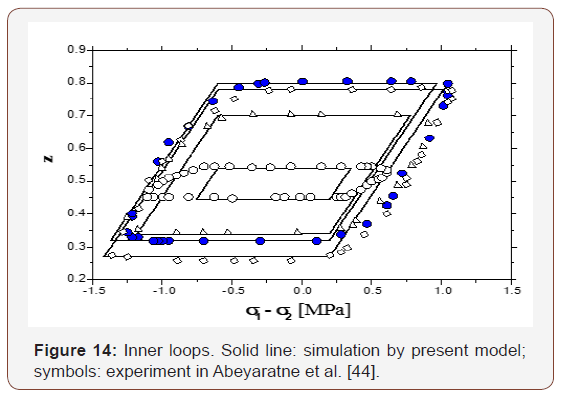For both speeds with Eqs. (54), (57), (58) & (59), one hasThis constant indicates that the sum of 1/2(ΠRe+- ΠRe)+ λ1 does not depend upon the cyclic speed. It is noticed that the present model is only applicable for the quasi-static case since the parameters Re ΠRe+, ΠRe and λ1 are expected to be material constants. For the non-quasi-static case, we have to consider the loading rate dependency for ΠRe+, and ΠRe and λ1 as well. It should be emphasized that all numerical simulation results and related figures in this paper are copied from JZ’s research report  with permission.

#### Conclusion

The model proposed in our previous paper  is applied to simulate the thermomechanical behavior of a single crystalline CuZnAl in the forward/reverse phase transformation and reorientation between martensite variants in a single crystalline CuAlNi. We assume that the interior hysteresis loop is controlled by the jump of the critical driving force when opposite transition happens. Our simulation for these two single crystal materials is compared with the experimental data. Good agreement is achieved.

It is found numerically that the variation of load device energy in the phase transformation is at the same level as the variation of stored energy in the austenite-martensite inter-phase. On the other hand, the energy stored in the interface among martensite variants is significantly smaller. The simulation also suggests that loading rate effect cannot be ignored in martensite reorientation at a high rate.

None.

#### Conflict of Interest

The author has no conflict of interest.

Article Details
Citation
Keywords
Scroll to
Scroll to Top